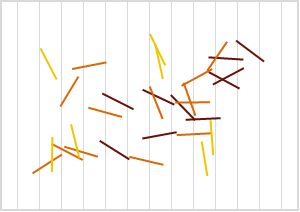# Throw a Two

Large tablecloth has parallel vertical lines unit apart. Thin wooden stick of length $\frac{3}{2}$ is twirled and tossed on the table. If $P$ is the chance that the stick crosses two lines when it lands and comes to rest, how much is $10^{10} \times P$, rounded to nearest whole number?

How large is the table exactly? Assume infinite expanse, infinitely thin lines, etc. The picture below depicts some sticks that are colored by the number of crossings.×